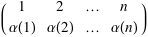AUTHOR PAGEMichael Sollami
Blog Posts from this author:
November 29, 2011–

Permutations are among the most basic elements of discrete mathematics. They are used to represent discrete groups of transformations, and in particular play a key role in group theory, the mathematical study of symmetry. Permutations and groups are important in many aspects of life. We all live on a giant sphere (the Earth) whose rotations are described by the group SO(3) (the special orthogonal group in 3 dimensions). On the micro-scale, the Hungarian-American physicist Eugene Wigner (November 17, 1902–January 1, 1995), who received a share of the Nobel Prize in Physics in 1963, discovered the “electron permutation group”, one of many applications of permutation groups to quantum mechanics.

Permutations deserve a full treatment in Wolfram|Alpha. Since Mathematica 8 provides new functionality to work with permutations using both list and cyclic representations, we now have a powerful new way of working with permutations using natural language.

Let’s first define permutations, then discuss how to work with them in Wolfram|Alpha. A permutation of a set X is a bijective (one-to-one and onto) mapping of X to itself. There is a convenient way of specifying a permutation α of a finite set of n elements: write down the numbers 1, 2, …, n in a row and write down their images under α in a row beneath:More »

February 21, 2011–

An algorithm is, in essence, a procedure given by a finite description that solves some computational problem. The field of computational complexity deals with questions of the efficiency of algorithms, i.e. “For a computational problem X, how many steps does the best algorithm perform in solving X?” You might think that questions in this field would be confined to the realm of computer science, except for the fact that computational complexity theory contains the mathematical problem of the century! Currently, many mathematicians around the world are attempting to solve the famed open problem P vs. NP, a problem so important that it is one of the seven millennium problems of the Clay Mathematics Institute and carries a million dollar prize. In fact, according to our logs, many of you tried to ask Wolfram|Alpha this same question before this new functionality was available! But before we talk about how Wolfram|Alpha can help you become a millionaire, let us begin with a historical overview of the subject. More »